# RD Sharma Solutions for Class 11 Chapter 1 - Sets Exercise 1.4

In Exercise 1.4 of Chapter 1, we shall discuss subsets and some results on subsets, universal set, power set. Students who aim to secure high marks in their board exams should refer to RD Sharma Class 11 Solutions which yield a good score. Solutions are designed by a team of experts to help students in their exam preparation. Students are advised to practice regularly, which improves logical thinking and problem-solving abilities as these are important aspects of learning. Students can download the pdf from the links provided below and can start practising offline.

## Download the Pdf of RD Sharma Solutions for Class 11 Maths Exercise 1.4 Chapter 1 – Sets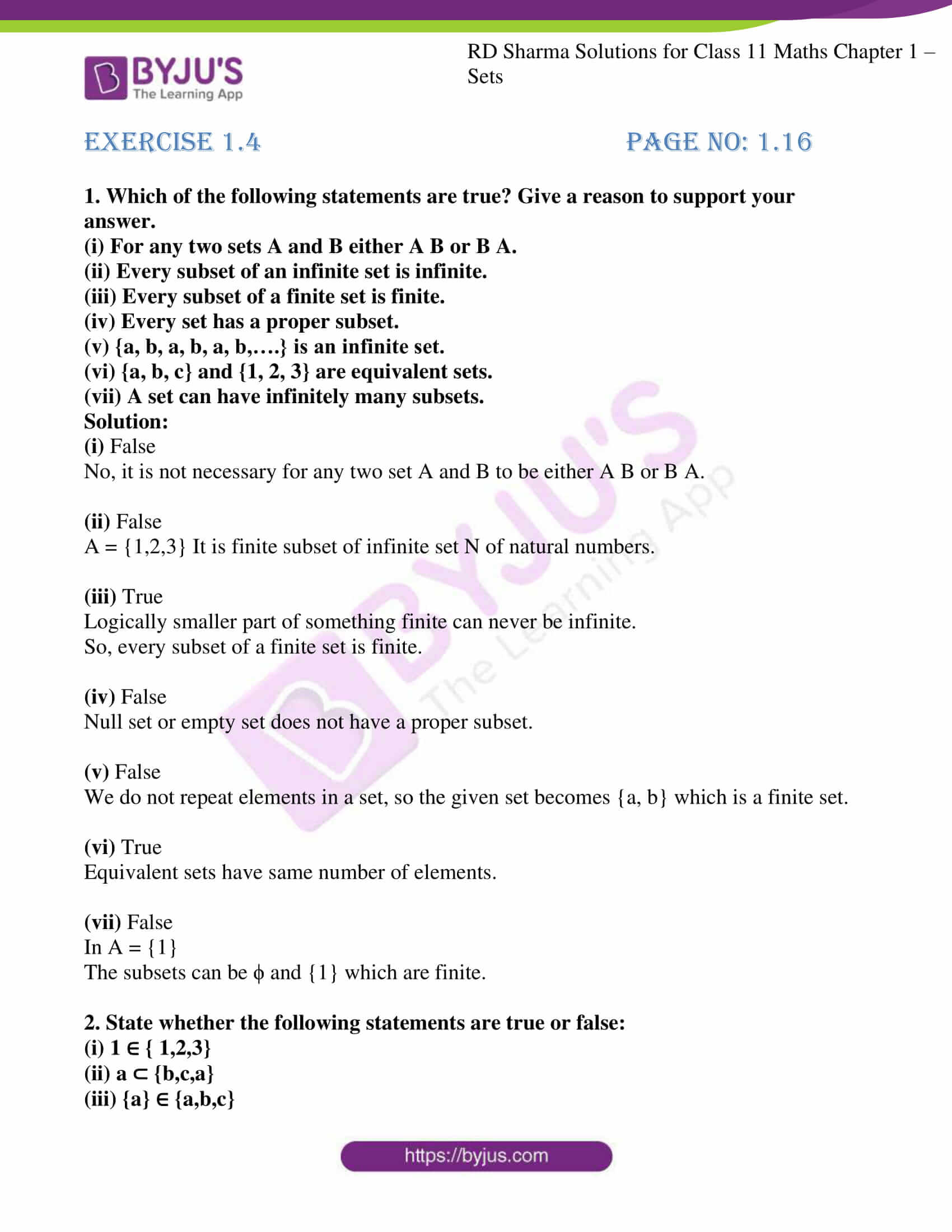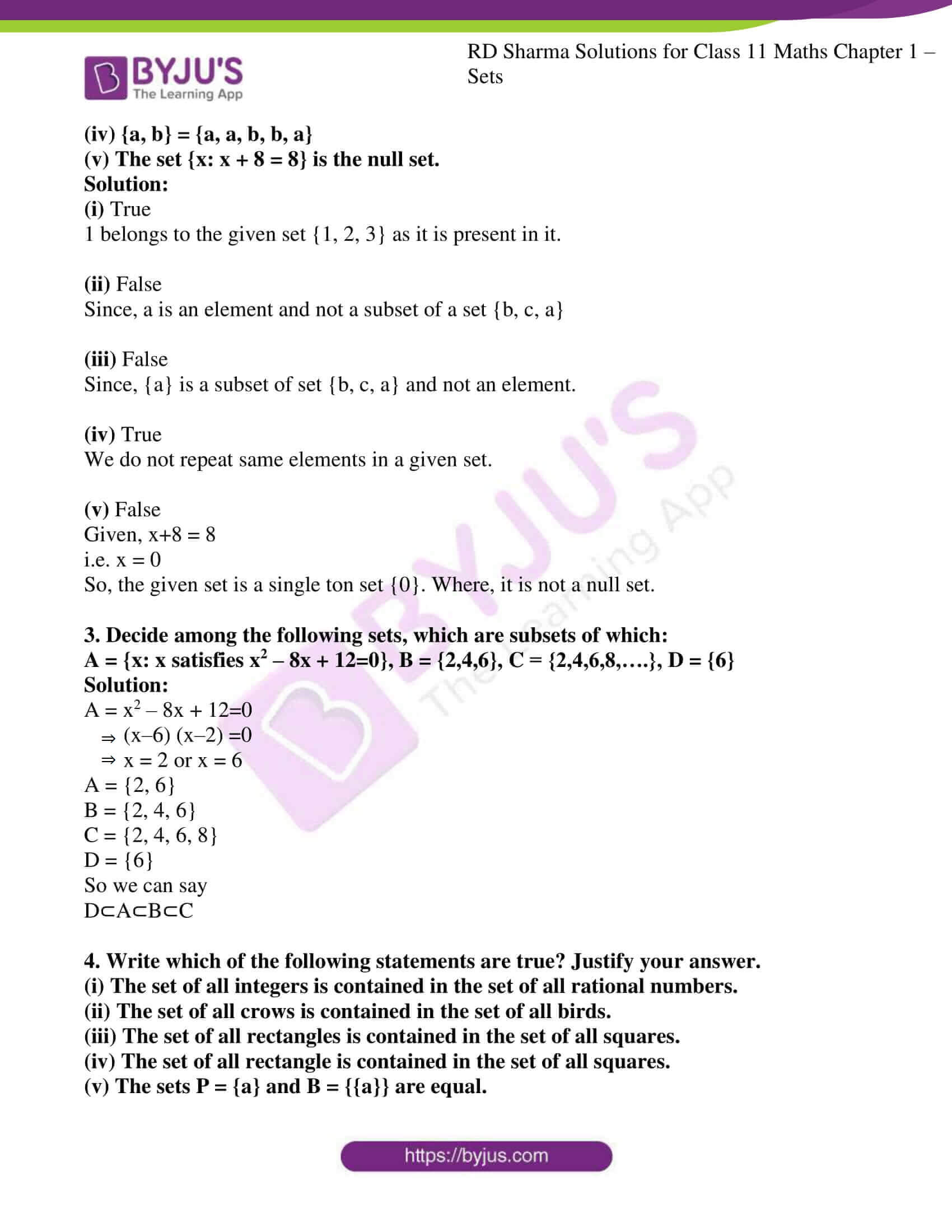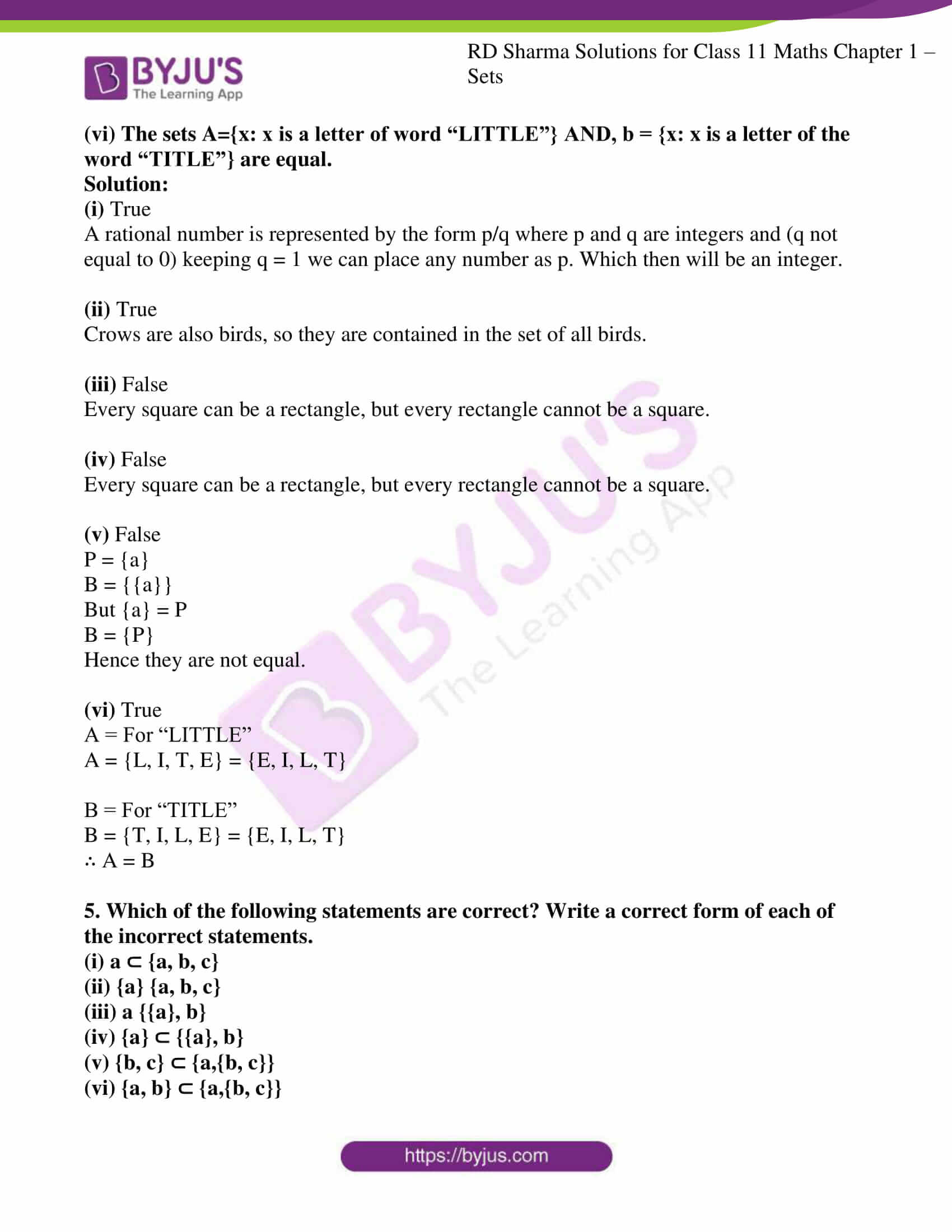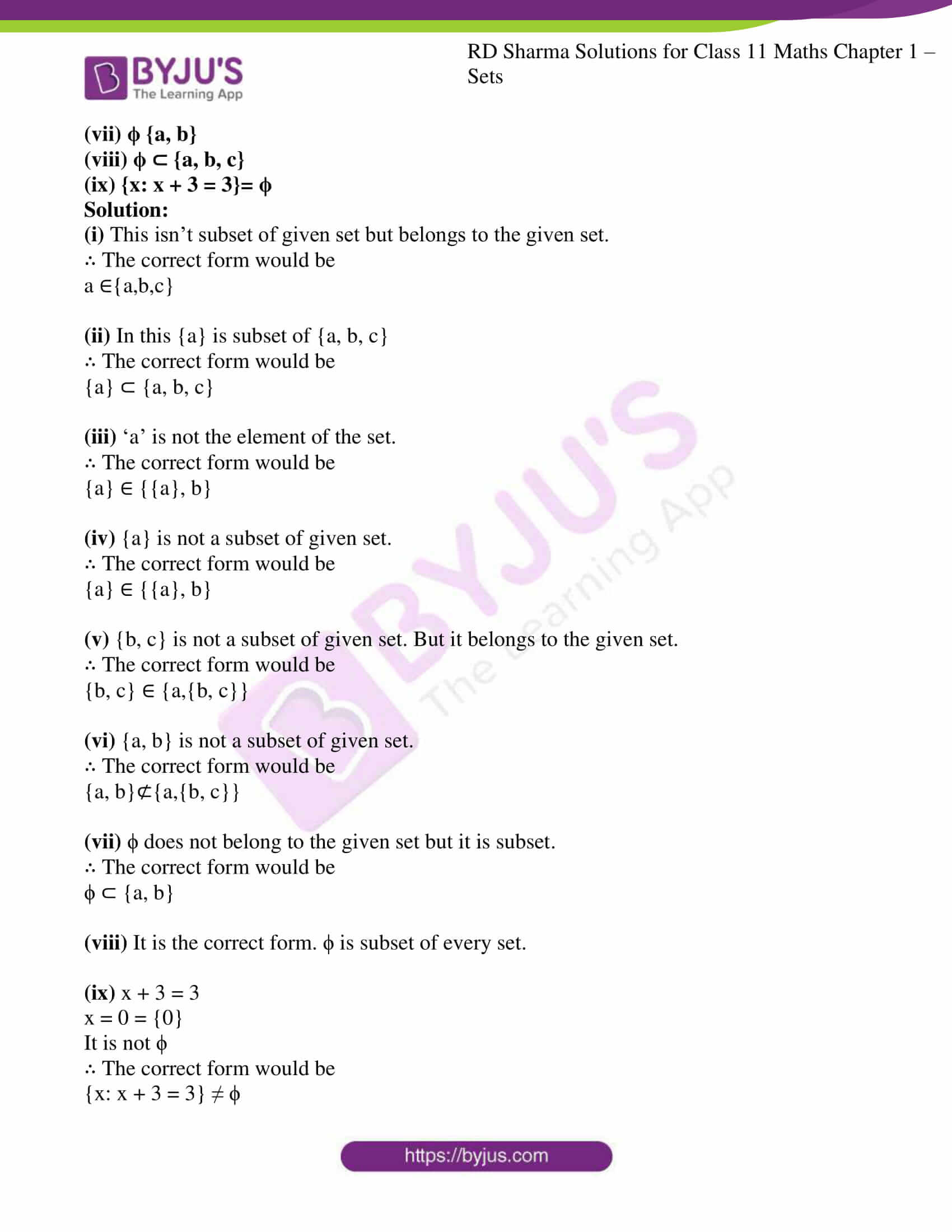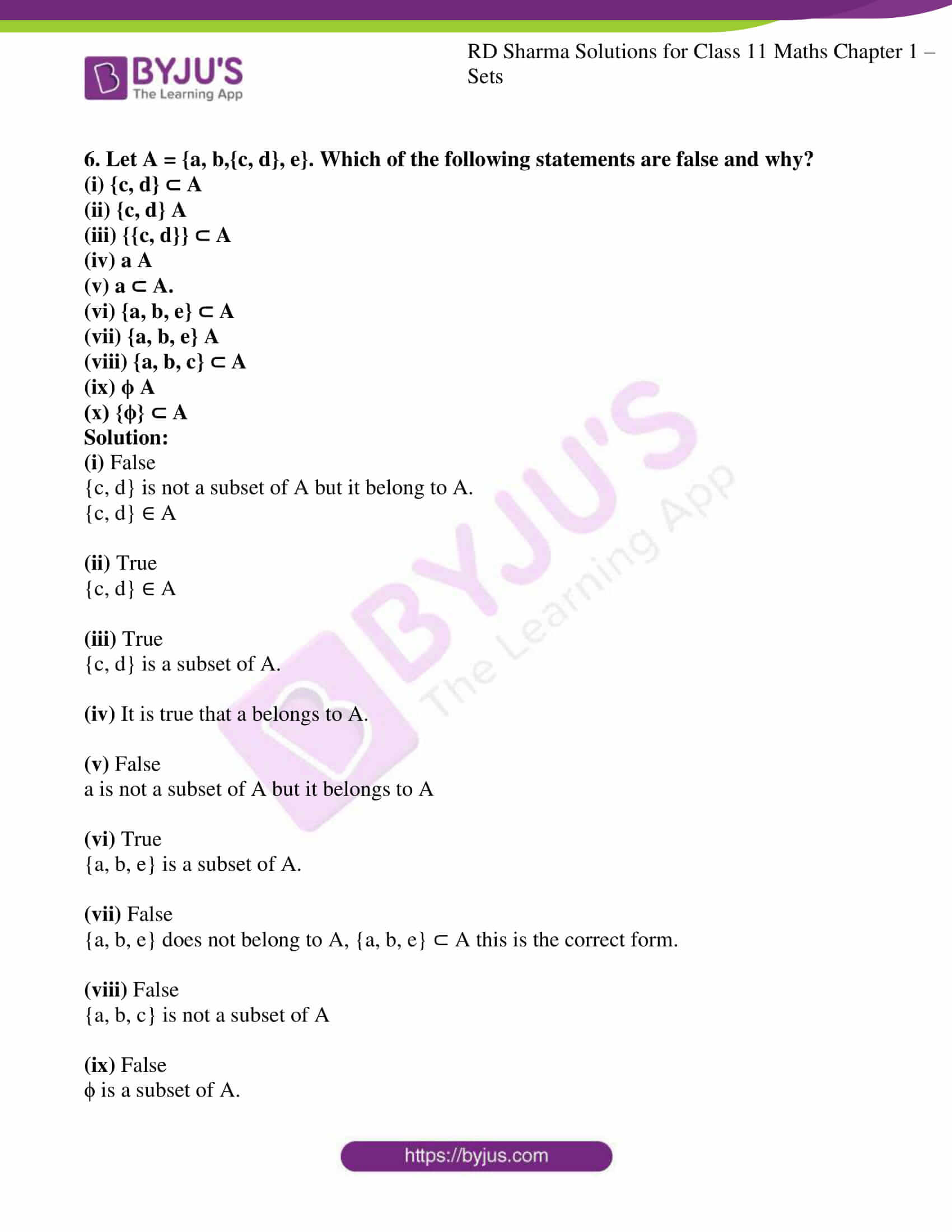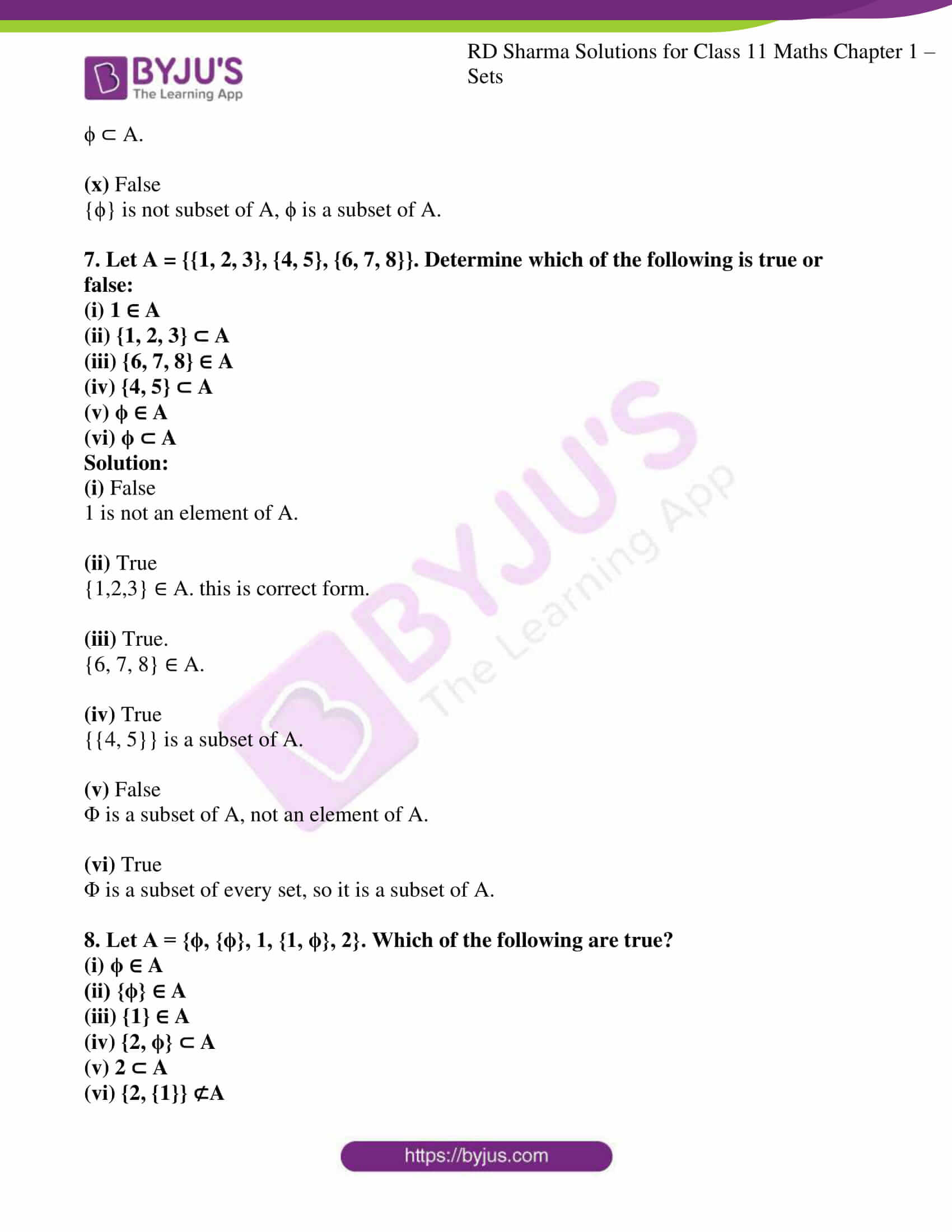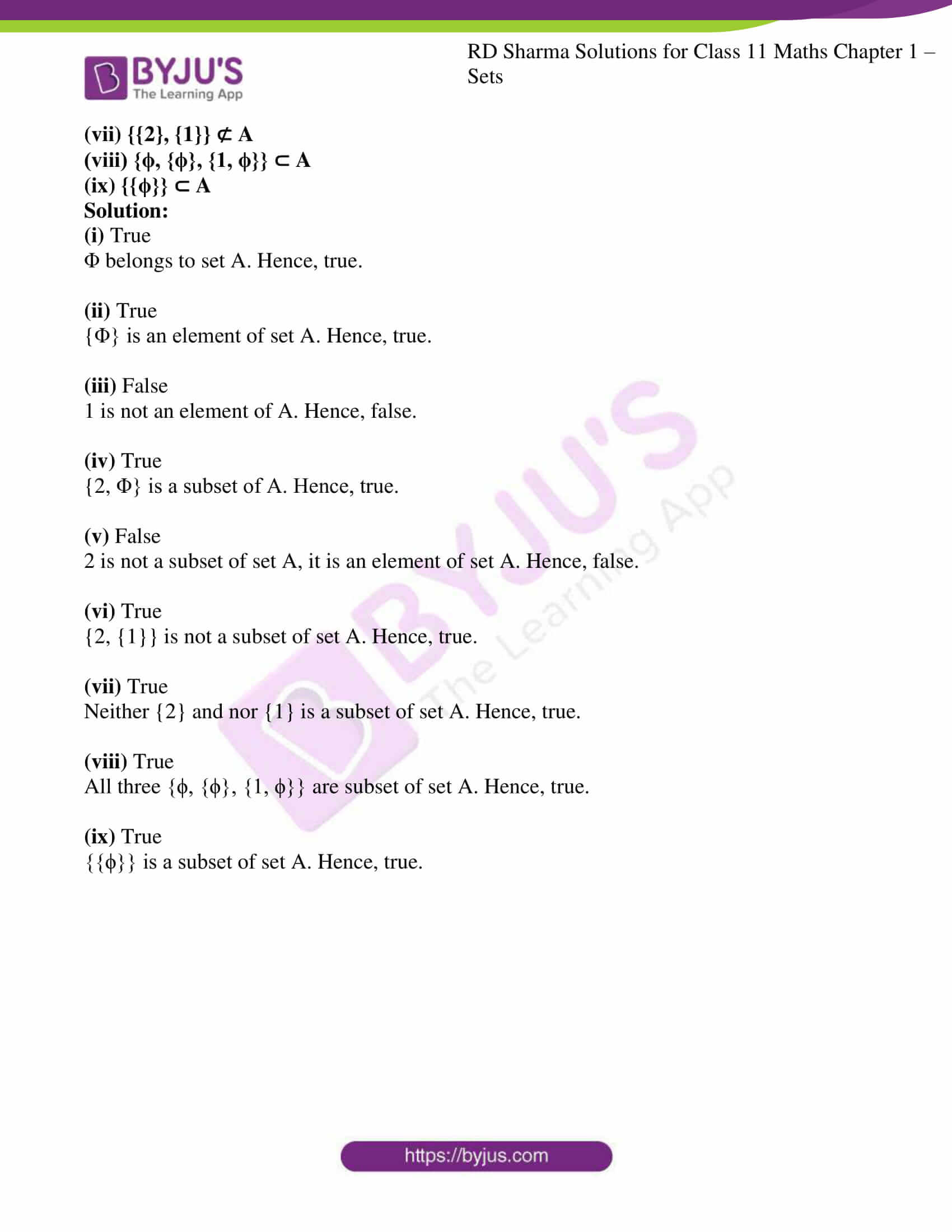### Access answers to RD Sharma Solutions for Class 11 Maths Exercise 1.4 Chapter 1 – Sets

1. Which of the following statements are true? Give a reason to support your answer.
(i) For any two sets A and B either A B or B A.
(ii) Every subset of an infinite set is infinite.
(iii) Every subset of a finite set is finite.
(iv) Every set has a proper subset.
(v) {a, b, a, b, a, b,….} is an infinite set.
(vi) {a, b, c} and {1, 2, 3} are equivalent sets.
(vii) A set can have infinitely many subsets.

Solution:

(i) False

No, it is not necessary for any two set A and B to be either A B or B A.

(ii) False

A = {1,2,3} It is finite subset of infinite set N of natural numbers.

(iii) True

Logically smaller part of something finite can never be infinite.

So, every subset of a finite set is finite.

(iv) False

Null set or empty set does not have a proper subset.

(v) False

We do not repeat elements in a set, so the given set becomes {a, b} which is a finite set.

(vi) True

Equivalent sets have same number of elements.

(vii) False

In A = {1}

The subsets can be ϕ and {1} which are finite.

2. State whether the following statements are true or false:
(i) 1 ∈ { 1,2,3}
(ii) a ⊂ {b,c,a}
(iii) {a} ∈ {a,b,c}
(iv) {a, b} = {a, a, b, b, a}
(v) The set {x: x + 8 = 8} is the null set.

Solution:

(i) True

1 belongs to the given set {1, 2, 3} as it is present in it.

(ii) False

Since, a is an element and not a subset of a set {b, c, a}

(iii) False

Since, {a} is a subset of set {b, c, a} and not an element.

(iv) True

We do not repeat same elements in a given set.

(v) False

Given, x+8 = 8

i.e. x = 0

So, the given set is a single ton set {0}. Where, it is not a null set.

3. Decide among the following sets, which are subsets of which:
A = {x: x satisfies x2 – 8x + 12=0}, B = {2,4,6}, C = {2,4,6,8,….}, D = {6}

Solution:

A = x2 – 8x + 12=0

⇒ (x–6) (x–2) =0

⇒ x = 2 or x = 6

A = {2, 6}

B = {2, 4, 6}

C = {2, 4, 6, 8}

D = {6}

So we can say

D⊂A⊂B⊂C

4. Write which of the following statements are true? Justify your answer.
(i) The set of all integers is contained in the set of all rational numbers.
(ii) The set of all crows is contained in the set of all birds.
(iii) The set of all rectangles is contained in the set of all squares.
(iv) The set of all rectangle is contained in the set of all squares.
(v) The sets P = {a} and B = {{a}} are equal.
(vi) The sets A={x: x is a letter of word “LITTLE”} AND, b = {x: x is a letter of the word “TITLE”} are equal.

Solution:

(i) True

A rational number is represented by the form p/q where p and q are integers and (q not equal to 0) keeping q = 1 we can place any number as p. Which then will be an integer.

(ii) True

Crows are also birds, so they are contained in the set of all birds.

(iii) False

Every square can be a rectangle, but every rectangle cannot be a square.

(iv) False

Every square can be a rectangle, but every rectangle cannot be a square.

(v) False

P = {a}

B = {{a}}

But {a} = P

B = {P}

Hence they are not equal.

(vi) True

A = For “LITTLE”

A = {L, I, T, E} = {E, I, L, T}

B = For “TITLE”

B = {T, I, L, E} = {E, I, L, T}

∴ A = B

5. Which of the following statements are correct? Write a correct form of each of the incorrect statements.
(i) a ⊂ {a, b, c}
(ii) {a} {a, b, c}
(iii) a {{a}, b}
(iv) {a} ⊂ {{a}, b}
(v) {b, c} ⊂ {a,{b, c}}
(vi) {a, b} ⊂ {a,{b, c}}
(vii) ϕ {a, b}
(viii) ϕ ⊂ {a, b, c}
(ix) {x: x + 3 = 3}= ϕ

Solution:

(i) This isn’t subset of given set but belongs to the given set.

∴ The correct form would be

a ∈{a,b,c}

(ii) In this {a} is subset of {a, b, c}

∴ The correct form would be

{a} ⊂ {a, b, c}

(iii) ‘a’ is not the element of the set.

∴ The correct form would be

{a} ∈ {{a}, b}

(iv) {a} is not a subset of given set.

∴ The correct form would be

{a} ∈ {{a}, b}

(v) {b, c} is not a subset of given set. But it belongs to the given set.

∴ The correct form would be

{b, c} ∈ {a,{b, c}}

(vi) {a, b} is not a subset of given set.

∴ The correct form would be

{a, b}⊄{a,{b, c}}

(vii) ϕ does not belong to the given set but it is subset.

∴ The correct form would be

ϕ ⊂ {a, b}

(viii) It is the correct form. ϕ is subset of every set.

(ix) x + 3 = 3

x = 0 = {0}

It is not ϕ

∴ The correct form would be

{x: x + 3 = 3} ≠ ϕ

6. Let A = {a, b,{c, d}, e}. Which of the following statements are false and why?
(i) {c, d} ⊂ A
(ii) {c, d} A
(iii) {{c, d}} ⊂ A
(iv) a A
(v) a ⊂ A.
(vi) {a, b, e} ⊂ A
(vii) {a, b, e} A
(viii) {a, b, c} ⊂ A
(ix) ϕ A
(x) {ϕ} ⊂ A

Solution:

(i) False

{c, d} is not a subset of A but it belong to A.

{c, d} ∈ A

(ii) True

{c, d} ∈ A

(iii) True

{c, d} is a subset of A.

(iv) It is true that a belongs to A.

(v) False

a is not a subset of A but it belongs to A

(vi) True

{a, b, e} is a subset of A.

(vii) False

{a, b, e} does not belong to A, {a, b, e} ⊂ A this is the correct form.

(viii) False

{a, b, c} is not a subset of A

(ix) False

ϕ is a subset of A.

ϕ ⊂ A.

(x) False

{ϕ} is not subset of A, ϕ is a subset of A.

7. Let A = {{1, 2, 3}, {4, 5}, {6, 7, 8}}. Determine which of the following is true or false:
(i) 1 ∈ A
(ii) {1, 2, 3} ⊂ A
(iii) {6, 7, 8} ∈ A
(iv) {4, 5} ⊂ A
(v) ϕ ∈ A
(vi) ϕ ⊂ A

Solution:

(i) False

1 is not an element of A.

(ii) True

{1,2,3} ∈ A. this is correct form.

(iii) True.

{6, 7, 8} ∈ A.

(iv) True

{{4, 5}} is a subset of A.

(v) False

Φ is a subset of A, not an element of A.

(vi) True

Φ is a subset of every set, so it is a subset of A.

8. Let A = {ϕ, {ϕ}, 1, {1, ϕ}, 2}. Which of the following are true?
(i) ϕ ∈ A
(ii) {ϕ} ∈ A
(iii) {1} ∈ A
(iv) {2, ϕ} ⊂ A
(v) 2 ⊂ A
(vi) {2, {1}} ⊄A
(vii) {{2}, {1}} ⊄ A
(viii) {ϕ, {ϕ}, {1, ϕ}} ⊂ A
(ix) {{ϕ}} ⊂ A

Solution:

(i) True

Φ belongs to set A. Hence, true.

(ii) True

{Φ} is an element of set A. Hence, true.

(iii) False

1 is not an element of A. Hence, false.

(iv) True

{2, Φ} is a subset of A. Hence, true.

(v) False

2 is not a subset of set A, it is an element of set A. Hence, false.

(vi) True

{2, {1}} is not a subset of set A. Hence, true.

(vii) True

Neither {2} and nor {1} is a subset of set A. Hence, true.

(viii) True

All three {ϕ, {ϕ}, {1, ϕ}} are subset of set A. Hence, true.

(ix) True

{{ϕ}} is a subset of set A. Hence, true.